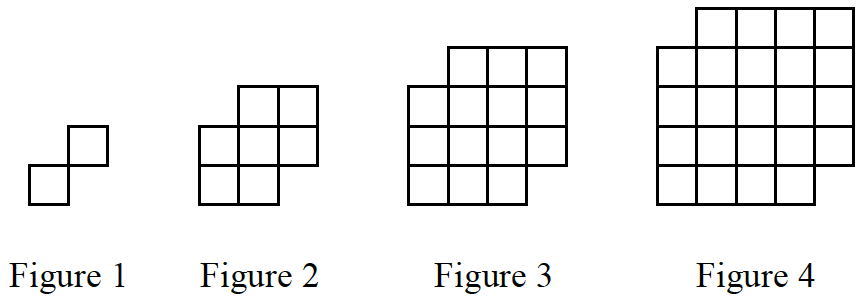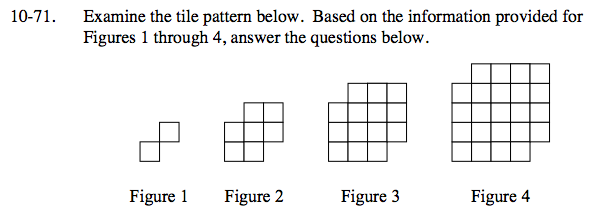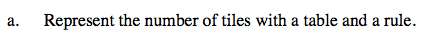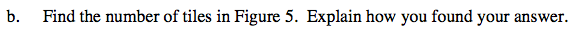### Home > CCA > Chapter 10 > Lesson 10.2.4 > Problem10-71

10-71.
1. Examine the tile pattern below. Based on the information provided for Figures 1 through 4, answer the questions below. Homework Help ✎1. Represent the number of tiles with a table and a rule.

2. Find the number of tiles in Figure 5. Explain how you found your answer.See problem 9-40.

You can use your table to write the rule.
If you are stuck, graph the points in your table.
What is the shape of your graph?

Using the tile pattern similar to problem 9-40:
y = x + (x − 1)(x + 1) + x
Using the table: y = (x + 1)2 − 2 = x2 + 2x − 1Use the pattern in your table or the equation from part (a).